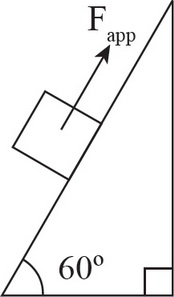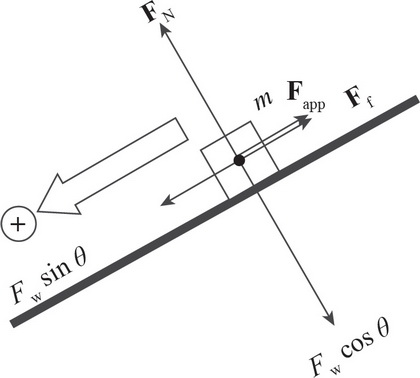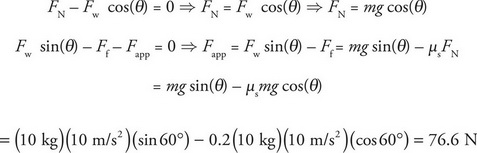# AP Physics 1 Question 428: Answer and Explanation

### Test Information

Question: 4286. A box with a mass of 10 kg is placed on an inclined plane that makes a 60° angle with the horizontal. The coefficient of static friction (μs) between the box and the inclined plane is 0.2. What force must be applied on the box in order to prevent the box from sliding down the inclined plane?

• A. 32.7 N
• B. 48.3 N
• C. 56.2 N
• D. 76.6 N

Explanation:

D

There are four forces acting on the box: the weight of the box, the normal force of the inclined plane on the box, the force applied, and the force of friction. The weight of the box can be separated into its parallel and perpendicular components.In order for the box to be at rest, the net force in both the parallel and perpendicular directions must be zero:A force of 76.6 N must be applied to prevent the box from sliding down the inclined plane.